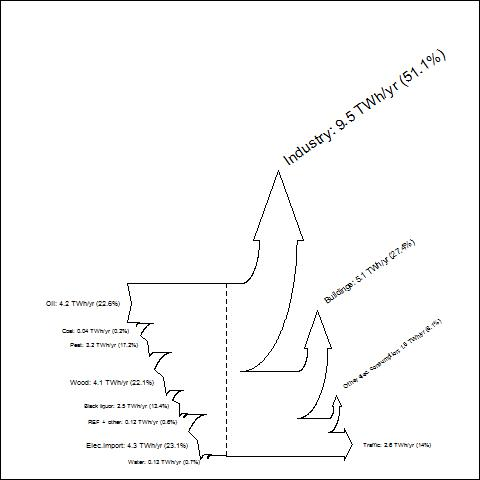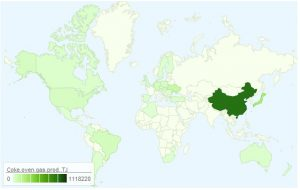# Visualize energy balance data

As energy engineer I have sometimes need to visualize energy balance data using Sankey diagram. In this example I will introduce how to do that with R.As a data source I will use  Central Finlands energy balance 2010 data.

Source of data you will find from this address: Keski-Suomen energiatase 2010

Ok let’s start to rock with R

To produce Sankey with R we use Function SankeyR (you will find same source as I use in this example from here)

We run first SankeyR function. Copy following code into your RGui.

```SankeyR <- function(inputs, losses, unit, labels, format="plot"){
########################
# SankeyR version 1.01 (updated August 10, 2010)
# is a function for creating Sankey Diagrams in R.
# See http://www.sankey-diagrams.com for excellent examples of Sankey Diagrams.
#
# OPTIONS:
# 'inputs' is a vector of input values
# 'losses' is a vector of loss values
# 'unit' is a string of the unit
# 'labels' is a vector of the labels for inputs and losses
# 'format' is the type of plotting:
#   The default is "plot," which produces a plot in the R graphics device.
#   Current alternate options include "pdf" and "bmp," which produce
#   those file types under the name "Sankey.xxx" in the current directory.
#
# Inputs do not need to equal losses.  Any difference will be displayed
# as a discrepancy in the height of the left and right sides of the diagram.
# This capability enables the developer to examine imbalances in flows.
# Percentages are a proportion of the inputs (so, the outputs might not equal 100%).
#
# EXAMPLE:
# Try using these values for the global carbon cycle, from Schlesinger (1997):
#  inputs = c(120,92)
#  losses = c(45,75,90,1,6)
#  unit = "GtC/yr"
#  labels = c("GPP","Ocean assimilation","Ra","Rh","Ocean loss","LULCC","Fossil fuel emissions")
#  SankeyR(inputs,losses,unit,labels)
#
# 8/10/10 - Added drawing for only one input.
#
# CREDITS:
# Created for R by Aaron BERDANIER
# send questions or comments to
# aaron.berdanier@gmail.com
#
# SankeyR is based strongly on drawSankey for Matlab,
# from James SPELLING, KTH-EGI-EKV (spelling@kth.se)
# http://leniwiki.epfl.ch/index.php/DrawSankey
#
# Licensees may copy, distribute, display, and perform the work and make
# derivative works based on it only for noncommercial purposes.
#
# Aaron would appreciate notification if you modify or improve this function.
########################```
```# Calculate fractional losses and inputs
frLosses = losses/sum(inputs)
frInputs = inputs/sum(inputs)```
```# First input and last output labels
inputLabel = paste(labels,": ",inputs," ",unit," (",round(100*frInputs,digits=1),"%)",sep="")
lossLabel = paste(labels[length(labels)],": ",losses[length(losses)]," ",unit,"
(",round(100*frLosses[length(losses)],digits=1),"%)",sep="")```
```########################
# Calculate position of plot axes (repeat of annotated code below; alternative: save values as vectors...)
limTop = frInputs; posTop = 0.4; maxy=0
limBot = 0; posBot = 0.1
if(length(inputs)>1){
for(j in 2:length(inputs)){
rI = max(0.07, abs(frInputs[j]/2))
rE = rI + abs(frInputs[j])
newPosB = posBot + rE*sin(pi/4) + 0.01
posBot = newPosB
arcEx = posBot - rE*sin(seq(0,pi/4,length.out=100))
arcEy = limBot - rE*(1-cos(seq(0,pi/4,length.out=100)))
arcIx = posBot - rI*sin(seq(0,pi/4,length.out=100))
arcIy = limBot - rE + rI*cos(seq(0,pi/4,length.out=100))
phiTip = pi/4 - 2*min(0.05, 0.8*abs(frInputs[j]))/(rI + rE)
xTip = posBot - (rE+rI)*sin(phiTip)/2
yTip = limBot - rE + (rE+rI)*cos(phiTip)/2
limBot = limBot - frInputs[j]
}
}else{}
posTop = posBot + 0.4
for(i in 1:(length(losses)-1)){
rI = max(0.07, abs(frLosses[i]/2))
rE = rI + abs(frLosses[i])
arcIx = posTop + rI*sin(seq(0,pi/2,length.out=100))
arcIy = limTop + rI*(1 - cos(seq(0,pi/2,length.out=100)))
arcEx = posTop + rE*sin(seq(0,pi/2,length.out=100));
arcEy = (limTop + rI) - rE*cos(seq(0,pi/2,length.out=100))
arEdge = max(0.015, rI/3)
arTop  = max(0.04, 0.8*frLosses[i])
arX = posTop + rI + c(0,-arEdge,frLosses[i]/2,frLosses[i]+arEdge,frLosses[i])
arY = limTop + rI + c(0,0,arTop,0,0)
if(max(arY)>maxy){maxy = max(arY)}else{maxy=maxy}
limTop = limTop - frLosses[i]
newPos = posTop + rE + 0.01
posTop = newPos
}
newPos = max(posTop, posBot) + max(0.05*limTop,0.05)
newPos = newPos + 0.8*(limTop-limBot)```
```maxx = newPos
miny = (limTop-frLosses[length(frLosses)])-max(0.015,abs(frLosses[length(frLosses)]/4))
maxy = maxy*2
minx = 0```
```########################
# Graphics type?
if(format!="plot"){
# Call graphics device
plottype = switch(format,
"pdf" = pdf("Sankey.pdf", width=11, height=min(8.5,11*(maxy-miny)/((maxx+3)-minx))),
"bmp" = bmp("Sankey.bmp", width=800*((maxx+3)-minx)/(maxy-miny), height=800, unit="px",res=144)
)
}```
```# Create plotting window
par(mar=c(0,0,0,0),oma=c(0,0,0,0))
plot(0,0,type="n",xlim=c(-1.5,maxx+1.5),ylim=c(miny,maxy),xaxt="n",yaxt="n")```
`w = 1 # line width`
```# Calculate fractional losses and inputs
frLosses = losses/sum(inputs)
frInputs = inputs/sum(inputs)```
```# Draw back edge of first input arrow
lines(c(0.1,0,0.05,0,0.4), c(0,0,frInputs/2,frInputs,frInputs),lwd=w)```
```# First input label
inputLabel = paste(labels,": ",inputs," ",unit," (",round(100*frInputs,digits=1),"%)",sep="")
fontsize = max(0.5,frInputs*2.5)
text(0, frInputs/2, inputLabel, cex=fontsize, pos=2) # try pos=4```
```# Set initial position for the top of the arrows
limTop = frInputs; posTop = 0.4; maxy=0```
```# set initial position for the bottom of the arrows
limBot = 0; posBot = 0.1```
```###
# DRAW ARROWS FOR ADDITIONAL INPUTS
if(length(inputs)>1){
for(j in 2:length(inputs)){```
```# determine inner and outer arrow radii
rI = max(0.07, abs(frInputs[j]/2))
rE = rI + abs(frInputs[j])
# push separation point forwards
newPosB = posBot + rE*sin(pi/4) + 0.01
lines(c(posBot,newPosB), c(limBot,limBot), lwd=w)
posBot = newPosB
# determine points on the external arc
arcEx = posBot - rE*sin(seq(0,pi/4,length.out=100))
arcEy = limBot - rE*(1-cos(seq(0,pi/4,length.out=100)))
# determine points on the internal arc
arcIx = posBot - rI*sin(seq(0,pi/4,length.out=100))
arcIy = limBot - rE + rI*cos(seq(0,pi/4,length.out=100))
# draw internal and external arcs
lines(arcIx, arcIy, lwd=w)
lines(arcEx, arcEy, lwd=w)```
```# determine arrow point tip
phiTip = pi/4 - 2*min(0.05, 0.8*abs(frInputs[j]))/(rI + rE)
xTip = posBot - (rE+rI)*sin(phiTip)/2
yTip = limBot - rE + (rE+rI)*cos(phiTip)/2
# draw back edge of additional input arrows
lines(c(min(arcEx),xTip,min(arcIx)), c(min(arcEy),yTip,min(arcIy)), lwd=w)```
```# Draw label
phiText = pi/2-2*min(0.05,0.8*abs(frInputs[j]))/(rI+rE)
xText = posBot-(rE+rI)*sin(phiText)/3
yText = limBot-rE/1.5+(rE+rI)*cos(phiText)/2
fullLabel = paste(labels[j],": ",inputs[j]," ",unit," (",round(100*frInputs[j],digits=1),"%)",sep="")
fontsize = max(0.5,frInputs[j]*2.5)
text(xText, yText, fullLabel, cex=fontsize, pos=2)```
```# save new bottom end of arrow
limBot = limBot - frInputs[j]
}```
`posTop = posBot + 0.4`
`lines(c(0.4,posTop), c(frInputs,frInputs), lwd=w)`
`lines(c(posBot,posBot+(posTop-posBot)/2), c(limBot,limBot),lwd=w)`
`posMid=posBot+(posTop-posBot)/2`
```}else{
lines(c(posBot,posBot+(posTop-posBot)/2), c(limBot,limBot),lwd=w)
posMid=posBot+(posTop-posBot)/2
}```
```###
# DRAW ARROWS OF LOSSES
for(i in 1:(length(losses)-1)){```
```# Determine inner and outer arrow radii
rI = max(0.07, abs(frLosses[i]/2))
rE = rI + abs(frLosses[i])
# Determine points on the internal arc
arcIx = posTop + rI*sin(seq(0,pi/2,length.out=100))
arcIy = limTop + rI*(1 - cos(seq(0,pi/2,length.out=100)))
# Determine points on the internal arc
arcEx = posTop + rE*sin(seq(0,pi/2,length.out=100));
arcEy = (limTop + rI) - rE*cos(seq(0,pi/2,length.out=100))
# Draw internal and external arcs
lines(arcIx, arcIy, lwd=w)
lines(arcEx, arcEy, lwd=w)```
```# Determine arrow tip dimensions
arEdge = max(0.015, rI/3)
arTop  = max(0.04, 0.8*frLosses[i])
# Determine points on arrow tip
arX = posTop + rI + c(0,-arEdge,frLosses[i]/2,frLosses[i]+arEdge,frLosses[i])
arY = limTop + rI + c(0,0,arTop,0,0)
if(max(arY)>maxy){maxy = max(arY)}else{maxy=maxy}
# Draw tip of losses arrow
lines(arX, arY, lwd=w)```
```# Draw label
txtX = posTop + rI + frLosses[i]/2
txtY = limTop + rI + arTop + 0.05
fullLabel = paste(labels[i+length(inputs)],": ",losses[i]," ",unit,"
(",round(100*frLosses[i],digits=1),"%)",sep="")
fontsize = max(0.5,frLosses[i]*2.5)
text(txtX, txtY, fullLabel, cex=fontsize, pos=4, srt=35)```
```# Save new position of arrow top
limTop = limTop - frLosses[i]
# Advance to new separation point
newPos = posTop + rE + 0.01
# Draw top line to new separation point
lines(c(posTop,newPos), c(limTop,limTop), lwd=w)
posTop = newPos```
`# SEPARATION LINES - not implemented yet`
`}`
```###
# Push the arrow forwards a little after all side-arrows drawn
newPos = max(posTop, posBot) + max(0.05*limTop,0.05)
# Draw lines to this new position
lines(c(posTop,newPos), c(limTop,limTop),lwd=w)
lines(c(posMid,newPos), c(limTop-frLosses[length(frLosses)],limTop-frLosses[length(frLosses)]),lwd=w)```
```# Draw final arrowhead for the output
lines(c(newPos,newPos,newPos+max(0.04,0.8*(frLosses[length(frLosses)])),newPos,newPos),
c(limTop,limTop+max(0.015,abs(frLosses[length```
```(frLosses)]/6)),limTop-frLosses[length(frLosses)]/2,(limTop-frLosses[length(frLosses)])-max(0.015,
abs(frLosses[length(frLosses)]/6)),(limTop-frLosses```
`[length(frLosses)])),  lwd=w)`
```# Save final tip position
newPos = newPos + 0.8*(frLosses[length(frLosses)])```
```# Last loss label
lossLabel = paste(labels[length(labels)],": ",losses[length(losses)]," ",unit,"
(",round(100*frLosses[length(losses)],digits=1),"%)",sep="")
fontsize = max(0.5,frLosses[length(losses)]*2.5)
text(newPos+0.05, limTop-frLosses[length(frLosses)]/2, lossLabel, cex=fontsize, pos=4) # try pos=4```
```# Draw mid-line
if(limBot<(limTop-frLosses[length(frLosses)])){
lines(c(posMid,posMid), c(frInputs,limBot),lty=2)
}else{
lines(c(posMid,posMid), c(frInputs,limTop-frLosses[length(frLosses)]),lty=2)
}```
```if(format!="plot"){
# Close graphics device
dev.off()
}}```

After that “Gui-run” we use Central Finlands energy balance data from year 2010…

```#Central Finlands energy balance 2010 (Keski-Suomen energiatase 2010 Source: http://www.kesto.fi)
#
# inputs
#Oil 4.2 TWh
#Coal 0,04
#Peat 3.2
#Wood fuel 4.1
#Black ? 2.5
#REF + other 0.12
#Water 0.13
#Elec.import 4.3```
```# lossess
#Industry 9.5
#--> Elec 4,65
#--> process heat 4,85```
```#Buildings 5.1
#--> district heating 2.55
#--> wood 0.663
#--> oil 0.969
#--> elec 0,867
#Other elec consumption 1.5
#Traffic 2.6
#--> Bensin  1.196
#--> Diesel 1.404
#total 18.6 TWh```
```inputs = c(4.2,0.04,3.2,4.1,2.5,0.12,4.3,0.13)
losses = c(9.5,5.1,1.5,2.6)
unit = "TWh/yr"
labels = c("Oil","Coal","Peat","Wood","Black liquor","REF + other","Elec.import","Water",
"Industry","Buildings", "Other elec. consumption", "Traffic")
SankeyR(inputs,losses,unit,labels)```
```#saving into file jpg. note: picture quality not so good
jpeg(".../energybalance.jpg")
SankeyR(inputs,losses,unit,labels)
dev.off()```
```#saving into file note: picture quality not so good
png(".../energybalance.png")
SankeyR(inputs,losses,unit,labels)
dev.off()```
```#saving into file note: note: picture quality not so good
bmp(".../energybalance.bmp")
SankeyR(inputs,losses,unit,labels)
dev.off()```
```#saving into file note: picture quality excellent
pdf(".../energybalance.pdf")
SankeyR(inputs,losses,unit,labels)
dev.off()```

Result is ok, but just now I have no idea how to produce “sub-branches” of losses. Anyway very nice routine to produce Sankey….And here you will find Sankey diagram in pdf format energybalance.

Have fun,
Marko

# Helppoa kuin heinäntekoHere in Finland we have phrase: ” Helppoa kuin heinänteko”. Now my purpose is to indicate what is the relation between this phrase and using R library googleVis.
In this example we use UN datasets from this source.

All datasets used in this examples You could download bloggerqvist server. Of course I encourage You to find appropriate UN dataset and find data within your interest.

## Example1: Coke Oven gas production 2006-2009 (TJ)

```################################################################ UN data source: http://data.un.org/ Coke oven gas - production ################################################################ # setting amazing library library(googleVis) ################################################################ # download data input <- read.table("http://energy.goeuropeinfo.com/data/un_data/UNdata_Export_20120622_210218958.csv", header=TRUE, sep=";", na.strings="NA", dec=",", strip.white=TRUE)```

```#selecting yearly data select09<- input[which(input\$Year=="2009"),] select08<- input[which(input\$Year=="2008"),] select07<- input[which(input\$Year=="2007"),] select06<- input[which(input\$Year=="2006"),] `````` #selecting variables Map09<- data.frame(select09\$Country.or.Area, select09\$Quantity) Map08<- data.frame(select08\$Country.or.Area, select08\$Quantity) Map07<- data.frame(select07\$Country.or.Area, select07\$Quantity) Map06<- data.frame(select06\$Country.or.Area, select06\$Quantity) `````` #change variable name names(Map09)<- c("Country", "Coke-oven gas prod. TJ") names(Map08)<- c("Country", "Coke-oven gas prod. TJ") names(Map07)<- c("Country", "Coke-oven gas prod. TJ") names(Map06)<- c("Country", "Coke-oven gas prod. TJ") `````` #year 2009 unmap09=gvisGeoMap(Map09, locationvar="Country", numvar="Coke-oven gas prod. TJ", options=list(height=350, dataMode='regions', chartid="Coke-oven prod 2009")) #year 2008 unmap08=gvisGeoMap(Map08, locationvar="Country", numvar="Coke-oven gas prod. TJ", options=list(height=350, dataMode='regions')) #year 2007 unmap07=gvisGeoMap(Map07, locationvar="Country", numvar="Coke-oven gas prod. TJ", options=list(height=350, dataMode='regions')) #year 2006 unmap06=gvisGeoMap(Map06, locationvar="Country", numvar="Coke-oven gas prod. TJ", options=list(height=350, dataMode='regions')) `````` #testing that all is ok plot(unmap09) plot(unmap08) plot(unmap07) plot(unmap06) `````` # saving just created map into html-file cat(unmap09\$html\$chart, file="j:/todo/UN/undata_cokeoven_prod_TJ_2009.html") cat(unmap08\$html\$chart, file="j:/todo/UN/undata_cokeoven_prod_TJ_2008.html") cat(unmap07\$html\$chart, file="j:/todo/UN/undata_cokeoven_prod_TJ_2007.html") cat(unmap06\$html\$chart, file="j:/todo/UN/undata_cokeoven_prod_TJ_2006.html")```

Results file looks like this:
Map 2009, 2008, 2007 and 2006

Have fun,
Marko

…..oops Here Is also an another example….

## Example2: Energy use (kg oil eqv per capita)```################################################################ UN data source: http://data.un.org/ energy use ################################################################ library(googleVis) input <- read.table("http://energy.goeuropeinfo.com/data/UNdata_Export_20120623_070334184_energyuse_kg_eqv_oil_per_capita.txt", header=TRUE, sep=";", na.strings="NA", dec=",", strip.white=TRUE) # select year into matrices select09<- input[which(input\$Year=="2009"),] select08<- input[which(input\$Year=="2008"),] select07<- input[which(input\$Year=="2007"),] select06<- input[which(input\$Year=="2006"),] `````` #select area and value field Map09<- data.frame(select09\$Country.or.Area, select09\$Value) Map08<- data.frame(select08\$Country.or.Area, select08\$Value) Map07<- data.frame(select07\$Country.or.Area, select07\$Value) Map06<- data.frame(select06\$Country.or.Area, select06\$Value) `````` names(Map09)<- c("Country", "Energy Use Eqv oil kg per capita") names(Map08)<- c("Country", "Energy Use Eqv oil kg per capita") names(Map07)<- c("Country", "Energy Use Eqv oil kg per capita") names(Map06)<- c("Country", "Energy Use Eqv oil kg per capita") `````` unmap09=gvisGeoMap(Map09, locationvar="Country", numvar="Energy Use Eqv oil kg per capita", options=list(height=350, dataMode='regions')) unmap08=gvisGeoMap(Map08, locationvar="Country", numvar="Energy Use Eqv oil kg per capita", options=list(height=350, dataMode='regions')) unmap07=gvisGeoMap(Map07, locationvar="Country", numvar="Energy Use Eqv oil kg per capita", options=list(height=350, dataMode='regions')) unmap06=gvisGeoMap(Map06, locationvar="Country", numvar="Energy Use Eqv oil kg per capita", options=list(height=350, dataMode='regions', chartid="06"),chartid="06") ```

plot(unmap09)
plot(unmap08)
plot(unmap07)
plot(unmap06)

``` cat(unmap09\$html\$chart, file="j:/todo/UN/undata_energyuse_eqvkgpercapita_2009.html") cat(unmap08\$html\$chart, file="j:/todo/UN/undata_energyuse_eqvkgpercapita_2008.html") cat(unmap07\$html\$chart, file="j:/todo/UN/undata_energyuse_eqvkgpercapita_2007.html") cat(unmap06\$html\$chart, file="j:/todo/UN/undata_energyuse_eqvkgpercapita_2006.html") ```

that’s it…